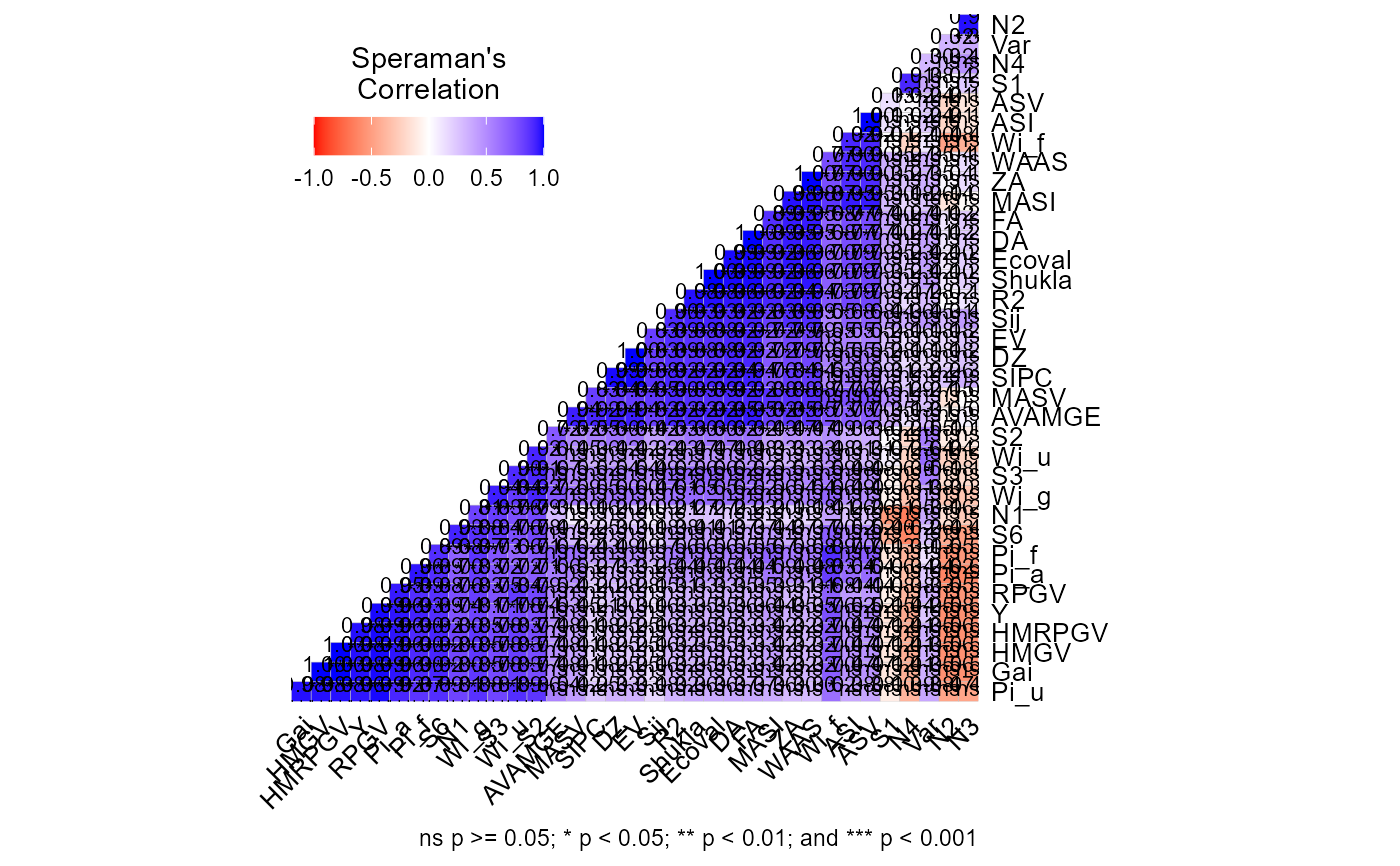Computes the Spearman's rank correlation between the parametric and nonparametric stability indexes computed with the function ge_stats().

## Usage

corr_stab_ind(x, stats = "all", plot = TRUE, ...)

## Arguments

x

An object of class ge_stats.

stats

The statistics to compute the correlation. See the section Details for more information.

plot

Plot the heat map with the correlations? Defaults to TRUE.

...

Other arguments to be passed to the function plot.corr_coef().

## Value

A list with the data (ranks) correlation, p-values and a heat map showing the correlation coefficients.

## Details

The argument stats is used to chose the statistics to show the ranks. Allowed values are "all" (All statistics, default), "par" (Parametric statistics), "nonpar" (Non-parametric statistics), "ammi" (AMMI-based stability statistics), or the following values that can be combined into comma-separated character vector. "Y" (Response variable), "Var" (Genotype's variance), "Shukla" (Shukla's variance), "Wi_g", "Wi_f", "Wi_u" (Annichiarrico's genotypic confidence index for all, favorable and unfavorable environments, respectively), "Ecoval" (Wricke's ecovalence), "Sij" (Deviations from the joint-regression analysis), "R2" (R-squared from the joint-regression analysis), "ASTAB" (AMMI Based Stability Parameter), "ASI" (AMMI Stability Index), "ASV" (AMMI-stability value), "AVAMGE" (Sum Across Environments of Absolute Value of GEI Modelled by AMMI ), "Da" (Annicchiarico's D Parameter values), "Dz" (Zhang's D Parameter), "EV" (Sums of the Averages of the Squared Eigenvector Values), "FA" (Stability Measure Based on Fitted AMMI Model), "MASV" (Modified AMMI Stability Value), "SIPC" (Sums of the Absolute Value of the IPC Scores), "Za" (Absolute Value of the Relative Contribution of IPCs to the Interaction), "WAAS" (Weighted average of absolute scores), "HMGV" (Harmonic mean of the genotypic value), "RPGV" (Relative performance of the genotypic values), "HMRPGV" (Harmonic mean of the relative performance of the genotypic values), "Pi_a", "Pi_f", "Pi_u" (Superiority indexes for all, favorable and unfavorable environments, respectively), "Gai" (Geometric adaptability index), "S1" (mean of the absolute rank differences of a genotype over the n environments), "S2" (variance among the ranks over the k environments), "S3" (sum of the absolute deviations), "S6" (relative sum of squares of rank for each genotype), "N1", "N2", "N3", "N4" (Thennarasu"s statistics)).

## Author

Tiago Olivoto tiagoolivoto@gmail.com

## Examples

# \donttest{
library(metan)
model <- ge_stats(data_ge, ENV, GEN, REP, GY)
#> Evaluating trait GY |============================================| 100% 00:00:20

a <- corr_stab_ind(model)# }